# KSEEB SSLC Class 10 Maths Solutions Chapter 3 Pair of Linear Equations in Two Variables Ex 3.4

In this chapter, we provide KSEEB SSLC Class 10 Maths Solutions Chapter 3 Pair of Linear Equations in Two Variables Ex 3.4 for English medium students, Which will very helpful for every student in their exams. Students can download the latest KSEEB SSLC Class 10 Maths Solutions Chapter 3 Pair of Linear Equations in Two Variables Ex 3.4 pdf, free KSEEB SSLC Class 10 Maths Solutions Chapter 3 Pair of Linear Equations in Two Variables Ex 3.4 book pdf download. Now you will get step by step solution to each question.

### Karnataka State Syllabus Class 10 Maths Chapter 3 Pair of Linear Equations in Two Variables Ex 3.4

Question 1.
Solve the following pair of linear equations by the elimination method and the substitution method :
(i) x + y = 5 and 2x – 3y = 4
(ii) 3x + 4y =10 and 2x – 2y = 2
(iii) 3x – 5y – 4 = 0 and 9x = 2y + 7
(iv) x2+2y3=−1 and x−y3=3
Solution:
(i) x + y = 5 and 2x – 3y = 4
x + y = 5 ………….. (i)
2x – 3y = 4 ………… (ii)
Multiplying eqn. (i) by 3
3x + 3y = 15 ………….. (iii)
By adding eqn. (ii) to eqn. (iii) ‘y’ is eliminated.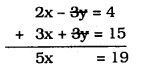∴ x=195
Substituting the value of ‘x’ in eqn. (i),
x + y = 5
195+y=5
y=51−195
=25−195
y=65
x=195y=65

(ii) 3x + 4y = 10 and 2x – 2y = 2
3x + 4y = 10 ……….. (i)
2x – 2y = 2 …………. (ii)
Multiplying eqn. (ii) by 2,
4x – 4y = 4 ………….. (iii)
Adding eqn. (i) to eqn. (ii),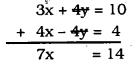∴ x=147=2
Substituting the value of ‘x’ in eqn. (i),
3x + 4y = 10
3 × 2 + 4y = 10
6 + 4y = 10
4y = 10 – 6
4y = 4
∴ y=44=1
∴ x = 2, y = 1.

(iii) 3x – 5y – 4 = 0 and 9x = 2y + 7
3x – 5y – 4 = 0 ⇒ 3x – 5y = 4 ……… (i)
9x = 2y + 7 ⇒ 9x – 2y = 7 ………. (ii)
Multiplying eqn. (i) by 3,
9x – 15y = 12 …………. (iii)
Subtracting eqn. (iii) from eqn. (ii)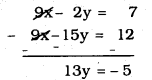∴ y=−513
Substituting the value of ‘y’ in eqn. (i),
3x – 5y = 4
3x−5(−513)=4
3x+2513=4 OR 3x=4−2513
3x=52−2513=2713
3x=2713
∴ x=2713×13
∴ x=913
∴ x=913y=−513

(iv) x2+2y3=−1 and x−y3=3
x2+2y3=−1×1−y3=3
3x+4y6=−13x−y3=3
3x + 4y = -6 ……….. (i) 3x – y = 9 …………… (ii)
Subtracting eqn. (ii) from eqn. (i),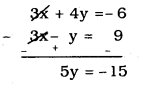y=−153=−3
y = -3
Substituting the value of ‘y’ in eqn. (i),
3x + 4y = -6
3x + 4 (-3) = -6
3x – 12 = -6
3x = -6 + 12
3x = 6
∴ x=63=2
∴ x = 2, y = 3

Question 2.
Form the pair of linear equations in the following problems, and find their solutions (if they exist) by the elimination method:

(i) If we add 1 to the numerator and subtract 1 from the denominator, a fraction reduces to 1. It becomes 12 if we only add 1 to the denominator. What is the fraction ?
Solution:
Let the fraction be xy, Here numerator is x and denominator is y.
∴ x+1y−1=1
x + 1 = y – 1
x – y = -1 – 1
x – y = -2 ……….. (i)
xy+1=12
2 × x = y + 1
2x = y + 1
2x – y = 1 ………. (ii)
Subtracting eqn. (ii) from eqn. (i),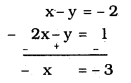∴ x = 3
Substituting the value of ‘x’ in eqn. (i),
x – y = -2
3 – y = -2
-y = -2 -3
-y = -5
∴ y = 5
∴ Fraction is xy=35

(ii) Five years ago, Nuri was thrice as old as Sonu. Ten years later, Nuri will be twice as old as Sonu. How old are Nuri and Sonu ?
Solution:
Present age of Nuri is ‘x’
Present age of Sonu is ‘y’
Five years ago, age of Nuri is x – 5
Five years ago, age of Sonu is y – 5
then, x – 5 = 3(y – 5)
x – 5 = 3y – 15
x – 3y = -15 + 5
x – 3y = -10 ……….. (i)
After 10 years, age of Nuri will be x + 10
After 10 years, age of Sonu will be y + 10
then, x +10 = 2(y + 10)
x + 10 = 2y + 20
x – 2y = 20 – 10
x – 2y = 10 …………. (ii)
Subtracting eqn. (ii) in eqn. (i),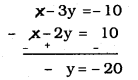∴ y = 20
Substituting the value of ‘y’ in eqn. (ii),
x – 2y = 10
x – 2(20) = 10
x – 40 = 10
x = 10 + 40
x = 50
∴ Present age of Nuri, x = 50
Present age of Sonu, y = 20.

(iii) The sum of the digits of a two-digit number is 9. Also, nine times this number is twice the number obtained by reversing the order of the digits. Find the number.
Solution:
Let the two digit number is 10x + y.
Sum of the digits of to digit number is
x + y = 9 ………….. (i)
Number obtained by reversing the order of the digits, we get 10y + x.
Twicing the 10y + x, it is nine times of first number.
∴ 2(10y + x) = 9(10x + 4)
20y + 2x = 90x + 9y
2x – 90x + 20y – 9y = 0
-88x + 11y = 0
88x – 11y = 0
8x – y = 0 ………….. (ii)
By adding eqn. (i) to eqn. (ii),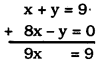∴ x=99=1
Substituting the value of ‘x’ in eqn. (i),
x + y = 9
1 + y = 9
y = 9 – 1
y = 8
∴ x = 1, y = 8
∴ Two digit number = 10x + y
= 10 × 1 + 8
= 10 + 8
=18.

(iv) Meena went to a bank to withdraw Rs. 2000. She asked the cashier to give her Rs. 50 and Rs. 100 notes only. Meena got 25 notes in all. Find how many notes of Rs. 50 and Rs. 100 she received.
Solution:
Let the Rs. 50 notes be ‘x’
Rs. 100 notes b ‘y’
x + y = 25 …………. (i)
x + 2y = 40 …………. (ii)
Subtracting eqn. (ii) from eqn. (i),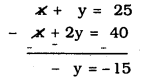∴ y = 15
Substituting the value of ‘y’ in eqn. (i)
x + y = 25
x+ 15 = 25
x = 25 – 15
∴ x = 10
∴ x = 10, y = 15
∴ Rs. 50’s notes are 10 and
Rs. 100’s notes are 15.

(v) A lending library has a fixed charge for the first three days and an additional charge for each day thereafter. Saritha paid Rs. 27 for a book kept for seven days, while Susy paid Rs. 21 for the book she kept for five days. Find the fixed charge and the charge for each extra day.
Solution:
Fixed charge be Rs. x.
x + 4y = 27 ………. (i)
x + 2y = 21 ………. (ii)
Subtracting Eqn. (ii) from eqn. (i),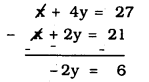∴ y=62=3
Substituting the value of ‘y’ in eqn. (i)
x + 4y = 27
x + 4(3) = 27
x + 12 = 27
∴ x = 27 – 12
∴ x = 15
∴ Fixed charge, x = Rs. 15.
Additional charge, y = Rs. 3.

All Chapter KSEEB Solutions For Class 10 Maths

—————————————————————————–

All Subject KSEEB Solutions For Class 10

*************************************************

I think you got complete solutions for this chapter. If You have any queries regarding this chapter, please comment on the below section our subject teacher will answer you. We tried our best to give complete solutions so you got good marks in your exam.

If these solutions have helped you, you can also share kseebsolutionsfor.com to your friends.

Best of Luck!!